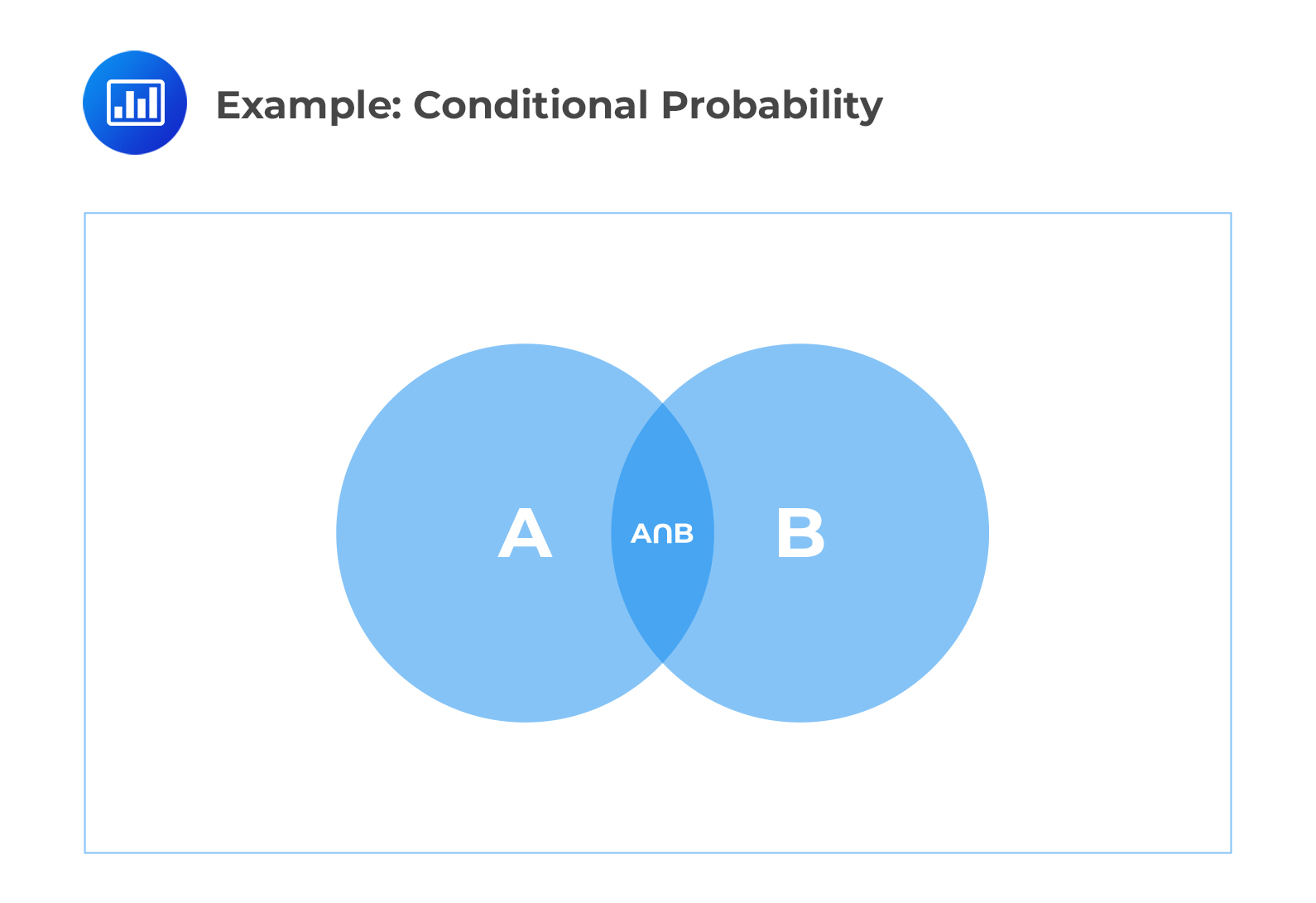Limited Time Offer: Save 10% on all 2022 Premium Study Packages with promo code: BLOG10# Define and calculate conditional probabilities

## Conditional Probability

Given two events $$A$$ and $$B$$, the conditional probability of event $$A$$ occurring, given that event $$B$$ has occurred, is the probability of event $$A$$ and $$B$$ occurring over the probability of event $$B$$ occurring as shown by the formula below:

$$P\left( A|B \right) =\frac { P\left( A\cap B \right) }{ P\left( B \right) }$$
as long as $$P (B) > 0$$.

As shown in the diagram below, the probability of $$A$$ given $$B$$ occurred is the shaded region $$A \cap B$$.

####Example: Conditional Probability

Suppose 50% of the students in a school take geometry, 30% take history, and 15% take both geometry and history. Given that a student selected at random takes geometry, what is the probability he or she also takes history?

Solution

Let event $$A$$ be “takes geometry” and event $$B$$ be “takes history.”

$$P \left(B \right) = 0.30$$

$$P \left(A \cap B \right) = 0.15$$

$$P\left( B|A \right) =\frac { P\left( A\cap B \right) }{ P\left( A \right) } ={ 0.15 }/{ 0.50 }=0.30\quad or\quad 30\%$$

This concept can be extended to more than 2 events as shown in the formula for 3 events below:

$$P\left( C|A\cap B \right) =\frac { P\left( A\cap B\cap C \right) }{ P\left( A \right) \times P\left( B|A \right) }$$

#### Example: Conditional Probability

Suppose that the students at a university are grouped such that:

45% take chemistry

35% take biology

25% take math

10% take chemistry and math

5% take chemistry and biology

3% take biology and math

1% take all three subjects

Calculate the probability that a student takes math, given that they take chemistry and biology.

$$P\left( \text{Math|Chemistry and Biology} \right) =\frac{{ P\left( \text{Math and Chemistry and Biology} \right) }}{{ P\left( \text{Chemistry} \right) \times P\left( \text{Biology|Chemistry} \right) }}$$

\begin{align*} P\left( \text{Biology|Chemistry} \right) & =\frac{{ P\left( \text{Biology and Chemistry} \right) }}{{ P\left( \text{Chemistry} \right) }} \\ & ={ .05 }/{ .45 }={ 1 }/{ 9 } \\ \end{align*}

$$P(\text{Math|Chemistry and Biology})=\frac{{ .01 }}{{ \left( { .45\times .05 }/{ .45 } \right) }}={ .01 }/{ .05 }={ 1 }/{ 5 }=.20\quad or\quad 20\%$$

The definition of conditional probability can be rewritten to come up with multiplication rule for probabilities:

$$P(A\cap B)=P(A|B)\bullet P(B)$$

Also, if the events A and B are independent, then:

$$P(A|B)=P(A)$$

Learning Outcome

Topic 1.e: General Probability – Define and calculate conditional probabilities.

Shop CFA® Exam Prep

Offered by AnalystPrepLevel I
Level II
Level III
All Three Levels
Featured Shop FRM® Exam PrepFRM Part I
FRM Part II
FRM Part I & Part II
Learn with Us

Subscribe to our newsletter and keep up with the latest and greatest tips for success
Shop Actuarial Exams PrepExam P (Probability)
Exam FM (Financial Mathematics)
Exams P & FM
Shop GMAT® Exam PrepComplete CourseDaniel Glyn
2021-03-24
I have finished my FRM1 thanks to AnalystPrep. And now using AnalystPrep for my FRM2 preparation. Professor Forjan is brilliant. He gives such good explanations and analogies. And more than anything makes learning fun. A big thank you to Analystprep and Professor Forjan. 5 stars all the way!michael walshe
2021-03-18
Professor James' videos are excellent for understanding the underlying theories behind financial engineering / financial analysis. The AnalystPrep videos were better than any of the others that I searched through on YouTube for providing a clear explanation of some concepts, such as Portfolio theory, CAPM, and Arbitrage Pricing theory. Watching these cleared up many of the unclarities I had in my head. Highly recommended.Nyka Smith
2021-02-18
Every concept is very well explained by Nilay Arun. kudos to you man!2021-02-13